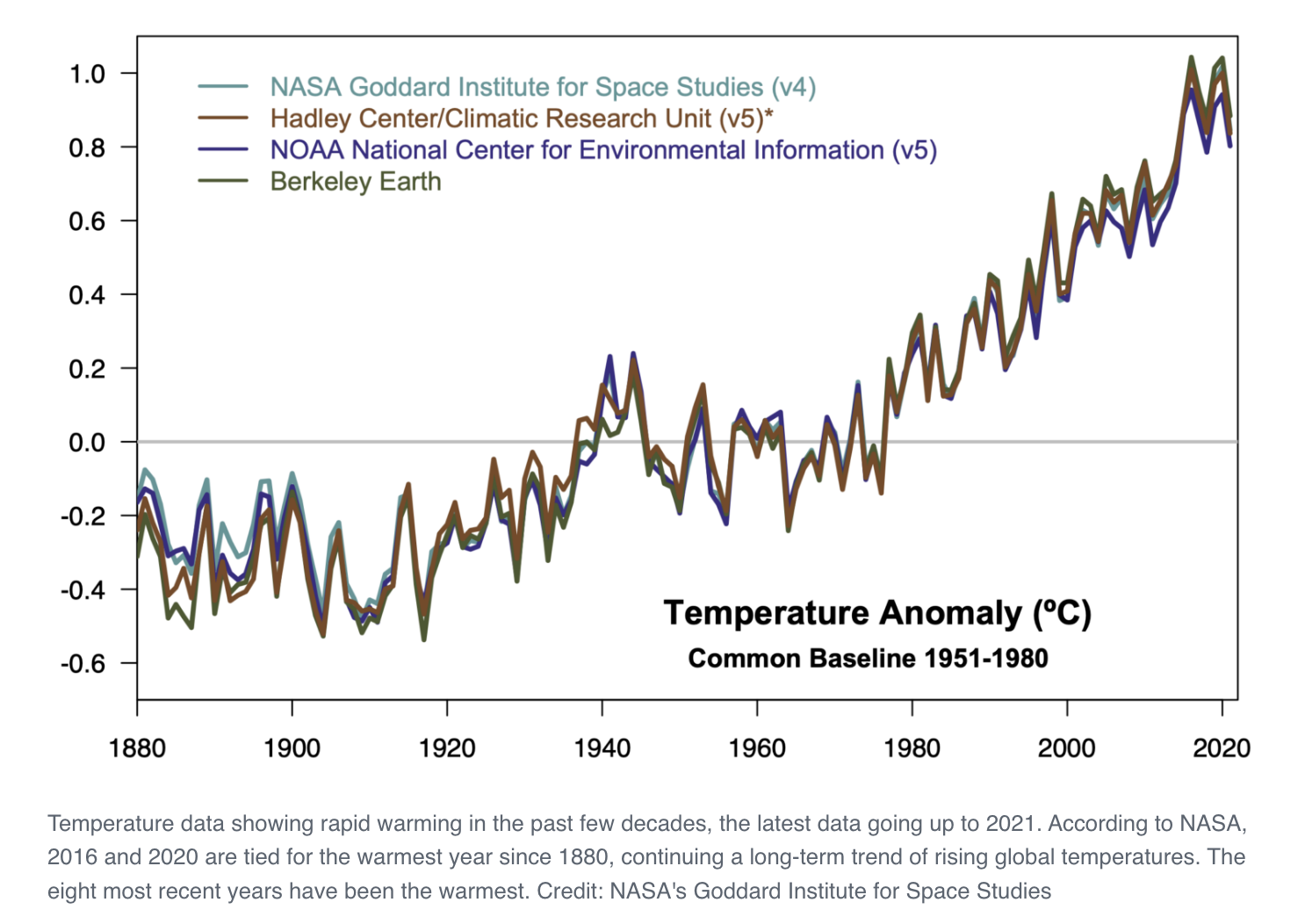# Using LSTM for time-series predictions

:

# Extra packages that we will use
# !pip install tensorflow

# Importing the required libraries
import pandas as pd
import numpy as np
import matplotlib.pyplot as plt
import tensorflow as tf
import seaborn as sns
import time
from sklearn.metrics import r2_score
from sklearn.metrics import mean_absolute_error , mean_squared_error
from sklearn.preprocessing import MinMaxScaler
from sklearn.preprocessing import LabelEncoder
from keras.callbacks import EarlyStopping
from keras.callbacks import ModelCheckpoint

from tensorflow.keras.layers import LSTM, Dense, Dropout, BatchNormalization
from tensorflow.keras.models import Sequential


There are three types of gates within a unit:

• Forget Gate: conditionally decides what information to throw away from the block.

• Input Gate: conditionally decides which values from the input to update the memory state.

• Output Gate: conditionally decides what to output based on input and the memory of the block.# LSTM Network

The Long Short-Term Memory network (LSTM) is a type of Recurrent Neural Network (RNN). A benefit of this type of network is that it can learn and remember over long sequences and does not rely on a pre-specified window lagged observation as input.

By default, an LSTM layer in Keras maintains state between data within one batch. A batch of data is a fixed-sized number of rows from the training dataset that defines how many patterns to process before updating the weights of the network. State in the LSTM layer between batches is cleared by default, therefore we must make the LSTM stateful. This gives us fine-grained control over when state of the LSTM layer is cleared, by calling the reset_states() function.

The LSTM layer expects input to be in a matrix with the dimensions: [samples, time steps, features].

• Samples: These are independent observations from the domain, typically rows of data.

• Time steps: These are separate time steps of a given variable for a given observation.

• Features: These are separate measures observed at the time of observation. We have some flexibility in how the dataset is framed for the network.